# Catch up on the latest AI articles# Does 2D GAN Know About 3D Shapes?

3 main points
✔️ Demonstrate that GANs implicitly learn 3D information
✔️ Proposes an unsupervised method recover 3D shapes from GANs trained on 2D images
✔️ Demonstrates superior performance compared to existing methods in 3D shape recovery and face image rotation

Do 2D GANs Know 3D Shape? Unsupervised 3D shape reconstruction from 2D Image GANs
written by Xingang PanBo DaiZiwei LiuChen Change LoyPing Luo
(Submitted on 2 Nov 2020 (v1), last revised 21 Feb 2021 (this version, v2))
Comments: Accepted to ICLR2021 oral.

Subjects: Computer Vision and Pattern Recognition (cs.CV)code：dataset：

## First of all

Generative Adversarial Networks (GANs) have shown very good performance in image generation and other applications, and have successfully generated a wide variety of data. In the paper presented in this article, we show that GANs trained with 2D images can also implicitly capture 3D information.

In other words, we have demonstrated that it is possible to recover the 3D shape of a 2D image from a GAN trained on the 2D image. The image is shown in the following figure.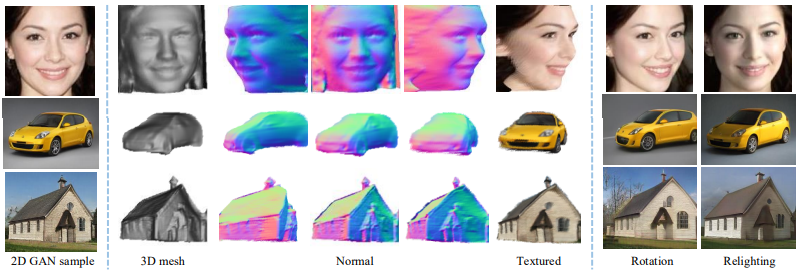The proposed framework is able to recover (unsupervised) 3D shapes from 2D images and also perform advanced operations such as changing the viewpoint (rotation) and changing the proof. Let's take a look at it below.

## Technique

The overall diagram of the proposed method is as follows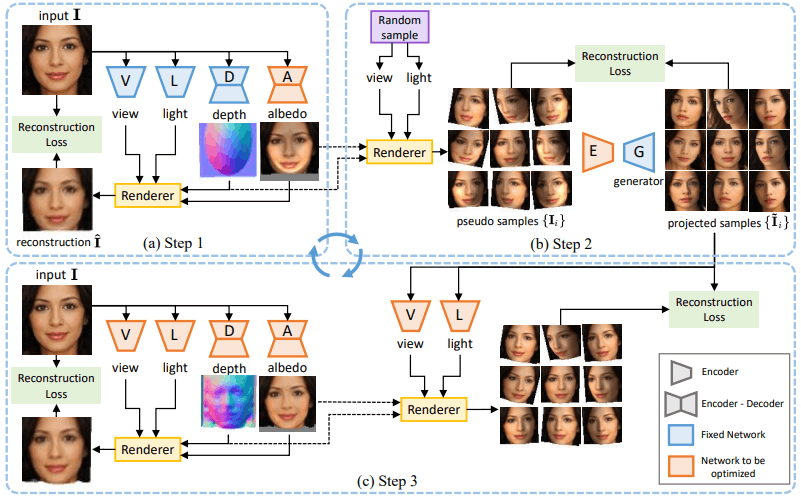This approach follows previous studies.

In this case, we take the image $I \in R^{3×H×W}$ as input, and use a function to predict four pieces of information consisting of the depth map $d \in R^{H×W}$ (depth information), the albedo (reflectivity) image $I \in R^{3×H×W}$, the viewpoint $v \in R^6$, and the direction of light $l \in S^2$. is used. As can be seen from the upper left (a) of the figure, this is predicted using the subnetworks ($D, A, V, L$) corresponding to the four pieces of information, respectively.

These four pieces of information are trained to recover the original input through a rendering process consisting of two steps, Lighting$\Lambda$ and Reprojection$\Pi$.

Lighting$\Lambda$ can be said to be an image that constructs a three-dimensional appearance based on the three-dimensional information (depth, reflectivity, and light direction) of the image, and reprojection$\Pi$ can be said to be an image that projects the appearance in three dimensions onto a two-dimensional image. The process of restoring the image through these and obtaining $\hat{I}$ can be summarized by the following equation.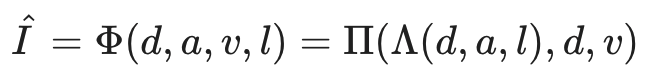This design follows previous studies as mentioned earlier, but while previous studies adopt symmetry of object shape as an assumption, our method avoids this assumption and better captures object asymmetry by the following procedure using GAN.

### Step 1 (Figure a): Use of weak shapes

Many objects, including faces and cars, are thought to have a slightly convex shape.

We then initialize the depth map $d$, corresponding to the image $I$, in the form of an ellipse, as shown in depth in Figure (a). We use the existing scene analysis model to position the ellipse so that it roughly matches the objects in the image.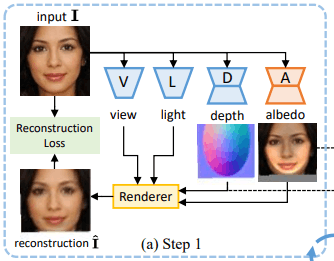Furthermore, we initialize the viewpoint as $v_0=0$ and the light direction as from the front and train the albedo network $A$ based on the reconstruction loss.

### Step 2: Sampling and projection onto GAN image manifold

The viewpoint $v$ and light direction $l$ is randomly sampled and rendered to obtain a pseudo-sample $\{I_i\}$.

As shown in the figure below, these pseudo-samples have unnatural distortions and shadows, but they also have information about the rotation of the face (corresponding to changes in viewpoint) and changes in light (corresponding to changes in light direction).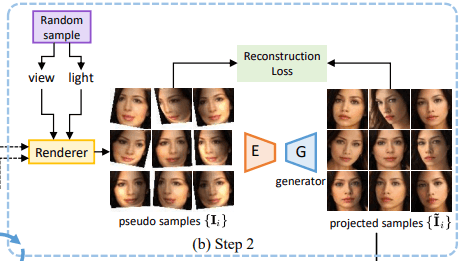Now, we reconstruct these pseudo-samples by GAN's Generator. Specifically, we train the encoder $E$ to predict the intermediate latent vector $w_i$ for each sample (without training the generator of GAN). In this case, the optimization objective is given by the following equation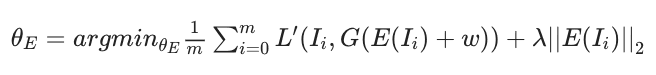where $m$ is the number of samples, $\theta_E$ is a parameter of the encoder $E$, $\lambda$ is the regularization factor, and $L^{\prime}$ is a measure of the image distance (L1 distance in the proposed method).

The $\lambda||E(I_i)||_2$ is a regularization term, which prevents the latent offset from becoming large (far outside the intermediate latent vector distribution) (a strong regularization method is also applied in the paper).

We use the Generator of GAN, but as mentioned earlier, we do not train the Generator. Therefore, even if there are unnatural distortions or shadows in the input image (which do not exist in normal 2D images and are not usually included in the output of GAN), such distortions or shadows will not appear in the generated result of the generator (as well as in the output of normal GAN).

Therefore, it is possible to generate natural images by correcting unnatural distortions and shadows that are not included in normal 2D images, while appropriately retaining information on the viewpoint, light changes, etc. of the pseudo-sample image.

### Step 3: Learning 3D shapes

The generated result (projection sample $\tilde{I}_i$) obtained in Step 2 can be said to be a successful change of viewpoint and light direction of the original image $I$.

In Step 3, we use this information to learn the 3D shape. Specifically, as shown in the figure below, the viewpoint and illumination network $V,L$ predicts the viewpoint and illumination direction $\tilde{v}_i,\tilde{l}_i$ for each sample $\tilde{I}$.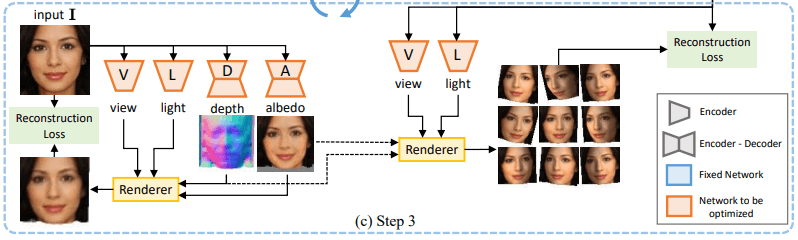The depth and albedo network $D,A$ outputs the depth and albedo images $\tilde{d},\tilde{a}$ with the original image $I$ as input. Rendering is performed based on these predictions, and training is performed so that this reconstructs each sample image. Here, the four networks are trained jointly by the following reconstruction objectives.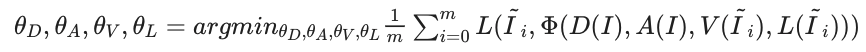In this case, not only the projection sample $tilde{I}_i$ generated in Step 2, but also the original image $I$ is used as one of the samples at the same time.

In addition, we randomly sampled the viewpoint and light direction $v_i,l_i$ for each sample in Step 2, but this is not used during training in Step 3 (because the viewpoint and light direction may have changed when generated by the GAN generator). By iterating through these Steps 1-3, we learn the 3D information appropriately (four iterations in our experiment).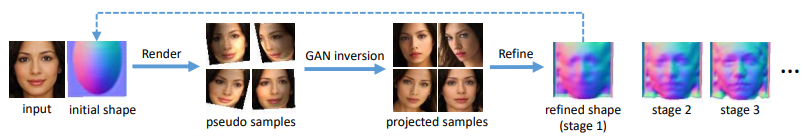In the discussion so far, we have assumed that the original image $I$ is a single image, but this can be extended to multiple images.

## experiment

In the experiments, we evaluate the proposed method for 3D shape restoration and then apply it to 3D image manipulation such as viewpoint change.

### Experiment setup

Data set

The dataset used was as follows

GAN Model

The GAN used within the proposed method is StyleGAN2, which has been pre-trained on the previously mentioned dataset.

### Unsupervised 3D Shape Reconstruction

Qualitative evaluation

The qualitative results of the proposed method and previous studies in Unsup3d are shown in the following figures.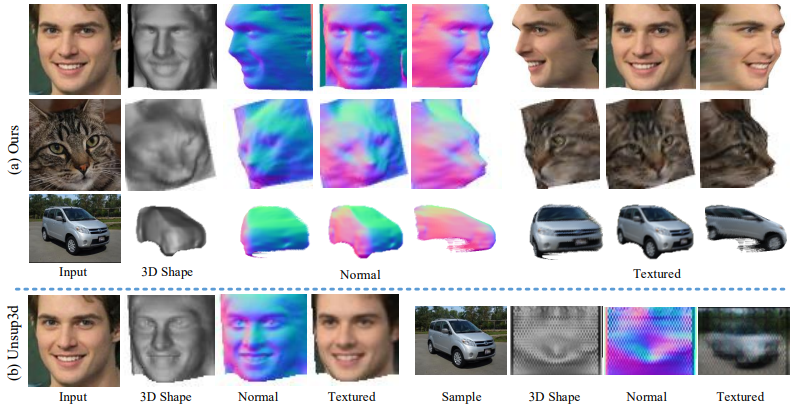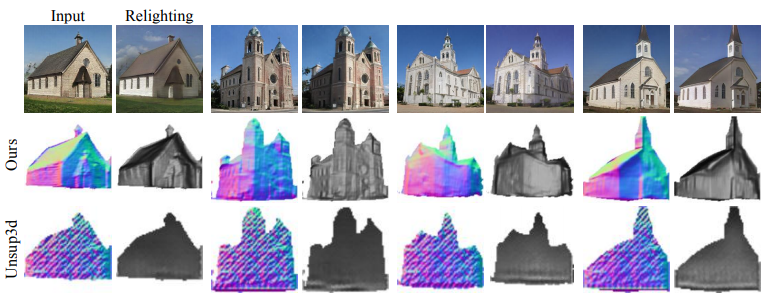As you can see in the figure, the 3D shapes of human faces, cats, cars, and buildings can be restored with high quality. The Unsup3d method also shows some good results, but it tends to perform poorly, especially for asymmetric objects such as cars and buildings.

Quantitative evaluation

For quantitative evaluation, we use the BFM dataset. Here, following previous studies, we use SIDE (scale-invariant depth error) and MAD (mean angle deviation) as indices for evaluation. The results are as follows.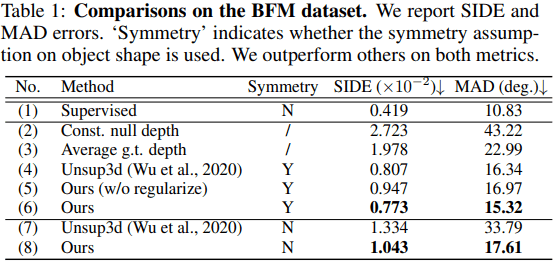Symmetry indicates the case with (Y) or without (N) the symmetry assumption. In general, the results outperform those of the previous study (Unsup3d) ((6),(8) in the table). Also, the case without latent offset regularization is shown in (5), which indicates that the regularization in the proposed method works effectively.

Furthermore, the proposed method used an ellipse to initialize the shape (depth map), but the comparison results when initialized with different settings are shown below.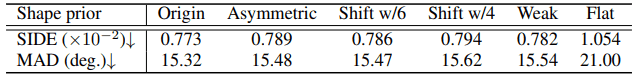Without going into details, we can see that the effect of the shape initialization setting on the performance is small. However, in the case of flat geometry (Flat), the performance is significantly worse, so it is necessary to initialize the 3D geometry to at least be able to capture changes in the viewpoint and light direction.

### On three-dimensional image manipulation

Rotation and Relighting of the object

After the training is completed, the proposed method can perform three-dimensional image manipulation by changing the viewpoint $v$ and light direction $l$ and rendering (or via encoder $E$ and GAN Generator$G$).

In the following figure, we show the result when the object is rotated and re-illuminated.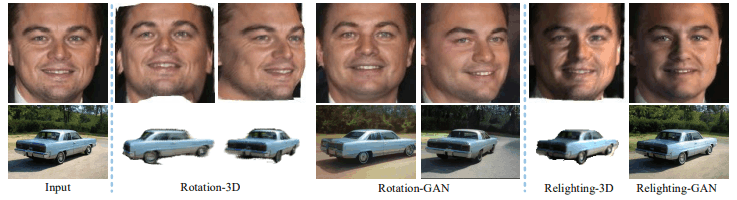Rotation(Relighting)-3D shows the case of rendering (from the restored 3D shape and albedo image), and Rotation(Relighting)-GAN shows the result of image generation via encoder-GAN. The rendered result faithfully reflects the structure of the object, and the GAN generates a very natural and realistic image, indicating that both are working effectively.

Face rotation to preserve Identity

We compare the proposed method with unsupervised methods (HoloGAN, GANSpace, and SeFa) that can perform face rotation using GAN.

Specifically, for each method, 100 random face images are rotated from -20 degrees to 20 degrees and 20 sample images are acquired. When using the general face identity detection model ArcFace, we evaluate how the face identity changes during the rotation. If the face image is properly rotated, the face identity is not expected to change significantly. The results are as follows.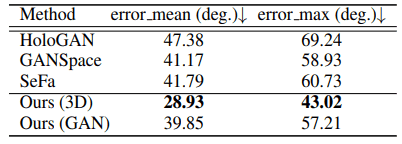As shown in the table, we can see that the loss is very small compared to the existing methods, and the face identity is effectively preserved. In addition, an actual generation example is shown below.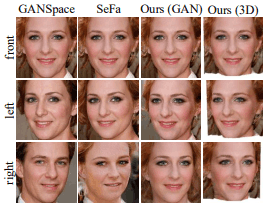In the existing method, the face changes significantly due to rotation (e.g., the gender changes in the right of GANSpace), whereas the proposed method (Ours) can recognize the same person.

### Future Issues

Although the proposed method worked very well, there were some cases where the 3D shape could not be recovered accurately, as shown in the following figure.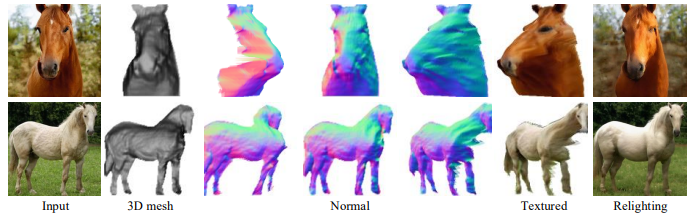This can be attributed to the fact that the initialization of the shape of the proposed method is a simple convex shape. In addition, since the 3D shape of the proposed method is parameterized by the depth map, the shape behind the object cannot be modeled. This may be solved by better parameterization such as handling the 3D mesh in some form other than the depth map, and future development is expected.

## summary

In the paper presented in this article, we proposed an innovative method for unsupervised 3D shape recovery from a GAN trained on 2D images. This not only provides an effective method for the 3D shape recovery task but also shows that GANs implicitly learn 3D information as well. GANs have been very successful in image generation, but now their potential is even more apparent.

If you have any suggestions for improvement of the content of the article,
please contact the AI-SCHOLAR editorial team through the contact form.